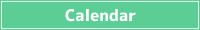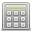<< < Oct. 2023 > >>
SunMonTueWedThuFriSat
1 2 3 4 5 6 7
8 9 10 11 12 13 14
15 16 17 18 19 20 21
22 23 24 25 26 27 28
29 30 31 1 2 3 4## Percentage Increase Calculator

You can do calculations such as how many is X percent increase of a number, or how many percentage points a number is over the original number.

What is the X percent increase in a number?

% increase of ?

By what percent is a number increased from the original number?

What % increase is from ?

What is the original number when there is an X percent increase?

If % increase of the original number is , what is the original number?

A percentage is a unit that expresses a percentage, and the whole is expressed as 100.

In the case of a percentage increase, the percentage after the increase is the original amount of 100 plus the percentage increase.

Original amount × (100 + Percentage increase) / 100 = Increased amount

For example, if you have a class of 50 people and the number increases by 20%, the class size after the increase will be 50 × (100 + 20) / 100 = 60 people.

An increase of 20 percent means that the original amount is 100 percent and it has become 120 percent. Because 120 / 100 = 1.2, it is the same as multiplying the original amount by 1.2.

## How to calculate percentage increase

### What is the X percent increase in a number?

If you want to calculate the X percent increase of some number, you can
Some Number × (100 + Percent Increase) / 100

Percentage represents the ratio of the original number to 100. So, by adding a percentage increase to 100 and dividing it by 100, you can find the ratio after the percentage increase when the original number is 1.

You can calculate X percent increase in a number by multiplying the number by the percentage.

### By what percent is a number increased from the original number?

If you want to calculate percentage increase of some number over original number, you can do it with
Some Number / Original Number × 100 - 100

A ratio is a value that represents the amount of something else relative to a source quantity, so you can find a ratio by dividing a number by the source number.

When expressed as a percentage, the whole is 100, so by multiplying that percentage by 100, you can calculate what percentage of the original number a certain number is.

If you want to calculate the percentage increase, subtract 100 from it to get the increase from the original number.

### What is the original number when there is an X percent increase?

If you want to calculate how many original numbers when you have a number with X percent increase, you can do it with
Some Number / (100 + Percent Increase) × 100

Since it is a number that increases by X percent from the original number, if you want to find the original number from a number, you can do the opposite by dividing it by the percentage.

The percentage is 100 of the total plus the percentage increase.

Since it's a percentage, you can calculate the original number by multiplying it by 100.

###Simple Calculator

Save results in a temporary